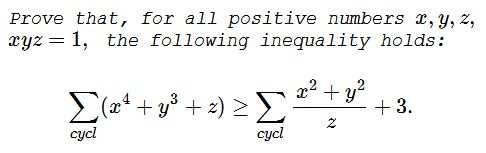# An Inequality with Cycling Sums

The following problem and its solution have been communicated to me by Dan Sitaru along with Proof 1. Proof 2 has been added by Imad Zak.### Proof 1

We use the two special cases of Schur's inequality:

$\displaystyle\begin{cases} t=1: & \sum_{cycl}x^3+3xyz\ge\sum_{cycl}xy(x+y),\\ t=2: & \sum_{cycl}x^4+xyz\sum_{cycl}x\ge\sum_{cycl}xy(x^2+y^2). \end{cases}$

The two inequalities are simplified by noting that $xyz=1.\;$ Add the two:

\displaystyle\begin{align} \sum_{cycl}(x^4+x^3+x)+3&\ge\sum_{cycl}\frac{x^2+y^2}{z}+\sum_{cycl}\left(\frac{x}{z}+\frac{y}{z}\right)\\ &=\sum_{cycl}\frac{x^2+y^2}{z}+\sum_{cycl}\left(\frac{x}{z}+\frac{z}{x}\right)\\ &\ge\sum_{cycl}\frac{x^2+y^2}{z}+6, \end{align}

which proves the required inequality.

### Proof 2

First note that by the AM-GM inequality, $\displaystyle\sum_{cycl}y^3\ge 3xyz=3.\;$ Thus (where we also used Schur's inequality with $t=2$),

\displaystyle\begin{align} \sum_{cycl}(x^4+y^3+z) &= \sum_{cycl}x^4+\sum_{cycl}y^3+\sum_{cycl}z\\ &\ge\sum_{cycl}x^4+\sum_{cycl}z+3\\ &=\sum_{cycl}x^4+xyz\sum_{cycl}x+3\\ &\ge\sum_{cycl}xy(x^2+y^2)+3\\ &=\sum_{cycl}\frac{1}{z}(x^2+y^2)+3. \end{align}

Equality holds when $x=y=z=1.$### Inequalities with the Product of Variables as a Constraint